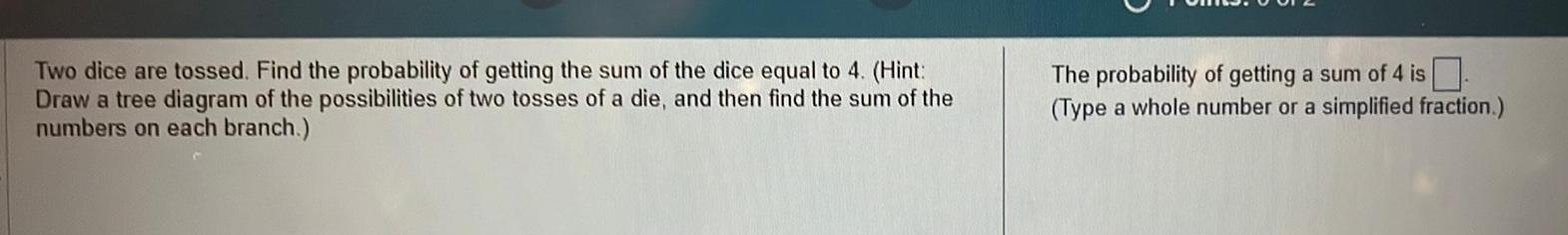Question:

# Two dice are tossed Find the probability of getting the sum

Last updated: 11/20/2023Two dice are tossed Find the probability of getting the sum of the dice equal to 4 Hint Draw a tree diagram of the possibilities of two tosses of a die and then find the sum of the numbers on each branch The probability of getting a sum of 4 is Type a whole number or a simplified fraction